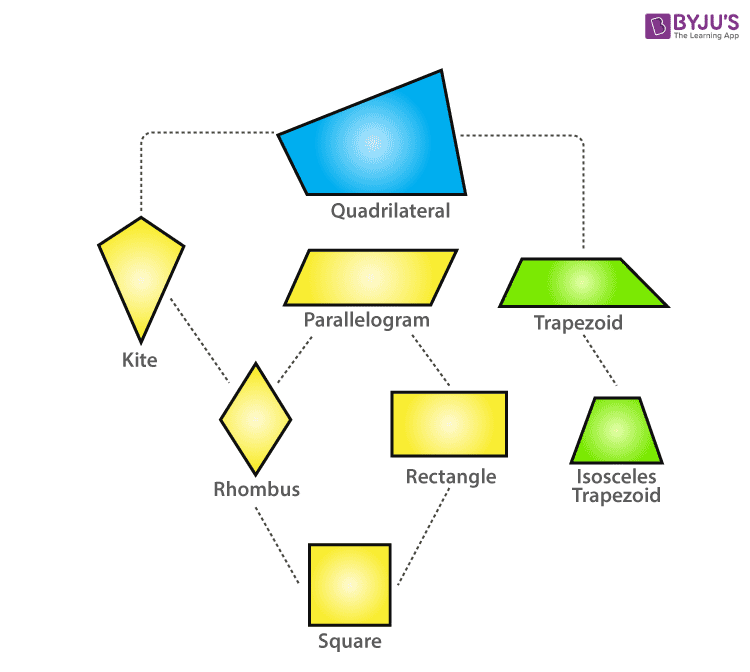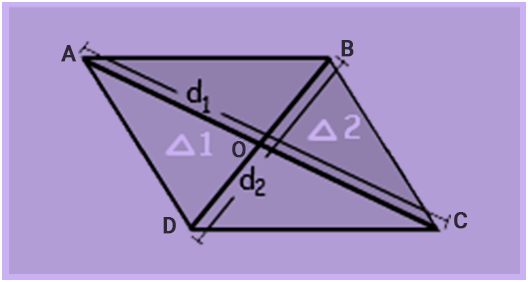Rhombus

Rhombus is a special case of a parallelogram. Before talking about the rhombus and its properties,  let us know what a quadrilateral is? A quadrilateral is a polygon containing 4 sides and 4 vertices enclosing 4 angles. The sum of the interior angles of a quadrilateral is equal to 360 degrees. The hierarchy of the quadrilateral is given as follows:Rhombus DefinitionA rhombus is a special case of a parallelogram and it is a four-sided quadrilateral. In a rhombus, opposite sides are parallel and opposite angles are equal. Moreover, all the sides of a rhombus are equal in length and the diagonals bisect each other at right angles. The rhombus is also called a diamond or rhombus diamond.

Rhombus Formulas

Area of Rhombus, A = (d1 x d2)/2 square units

The perimeter of Rhombus, P = 4a units

Where, the diagonals of the rhombus are d1 and d2

‘a’ is the side

Properties of Rhombus

Some of the important properties of the rhombus are as follows:

• All sides of the rhombus are equal.
• The opposite sides of a rhombus are parallel.
• Opposite angles of a rhombus are equal.
• In a rhombus, diagonals bisecting each other at right angles.
• Diagonals bisect the angles of a rhombus.
• The sum of two adjacent angles is equal to 180 degrees.
• The two diagonals of a rhombus form four right-angled triangles which are congruent to each other
• You will get a rectangle when you join the midpoint of the sides.
• You will get another rhombus when you join the midpoints of half the diagonal.
• Around a rhombus, there can be no circumscribing circle.
• Within a rhombus, there can be no inscribing circle.
• You will get a rectangle, where the midpoints of the 4 sides are joined together and the length and width of the rectangle will be half the value of the main diagonal. So that the area of the rectangle will be half of the rhombus.
• When the shorter diagonal is equal to one of the sides of a rhombus, two congruent equilateral triangles are formed.
• You will get a cylindrical surface having a convex cone at one end and concave cone at another end when the rhombus is revolved about any side as the axis of rotation
• You will get a cylindrical surface having concave cones on both the ends when the rhombus is revolved about the line joining the midpoints of the opposite sides as the axis of rotation
• You will get a solid with two cones attached to their bases when the rhombus is revolving about the longer diagonal as the axis of rotation. In this case, the maximum diameter of the solid is equal to the shorter diagonal of the rhombus.
• You will get a solid with two cones attached to their bases when the rhombus is revolving about the shorter diagonal as the axis of rotation. In this case, the maximum diameter of the solid is equal to the longer diagonal of the rhombus.

Examples

The sample example for the rhombus is given below.

Question:

The two diagonal lengths d1 and d2 of a rhombus are 6cm and 12 cm respectively. Find its area.

Solution:

Given:

Diagonal d1 = 6cm

Diagonal d2= 12 cm

Area of the rhombus, A = (d1 x d2)/2 square units

A = ( 6 x 12)/2

A = 72/2

A = 36 cm2

Therefore, the area of rhombus = 36 square units.

Question 2: Find the diagonal of a rhombus if its area is 121 cm2 and length measure of longest diagonal is 22 cm.

Solution:

Given: Area of rhombus = 121 cm2 and Lets say d1 = 22 cm.

Using Area of the rhombus formula,  A = (d1 x d2)/2 square units, we get

121 = (22 x d2)/2

121 = 11 x d2

or  11 =  d2

For more such interesting information on properties of the quadrilateral, register with BYJU’S – The Learning App and also watch videos to learn with ease.